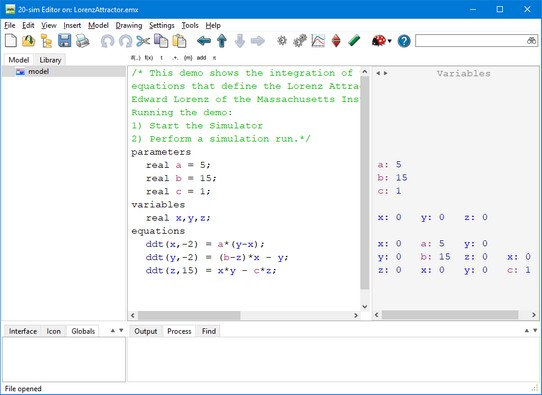﻿ 20-sim webhelp > Editor > Introduction > Equation Editor

Equation Editor

Equations models are the models at the lowest level in the model hierarchy. A model without an interface (inputs, output, ports), will have no hierarchy and is thus automatically an equation model. If you have opened an equation model, the right part of the Editor shows the Equation Editor. In the Equation Editor you can enter and edit equation models.Equation model with the Equation Editor (right part).

Use

Put your mouse in the Equation Editor and start typing. If you click on the buttons of the taskbar, sample code is inserted. Read the language reference section to find out more.

Color Syntax Highlighting

Functions, Variables etc. are given special colors to distiguish them from the other text.

Auto Indent

If a line is indented (using the tab), the next line will start at the same indentation. You can remove indentation by clicking the Backspace button.

Multi-Line Tabbing

You can give multiple lines of code a new indentation by clicking the tab button. Select all the lines and click Shift-tab to remove.